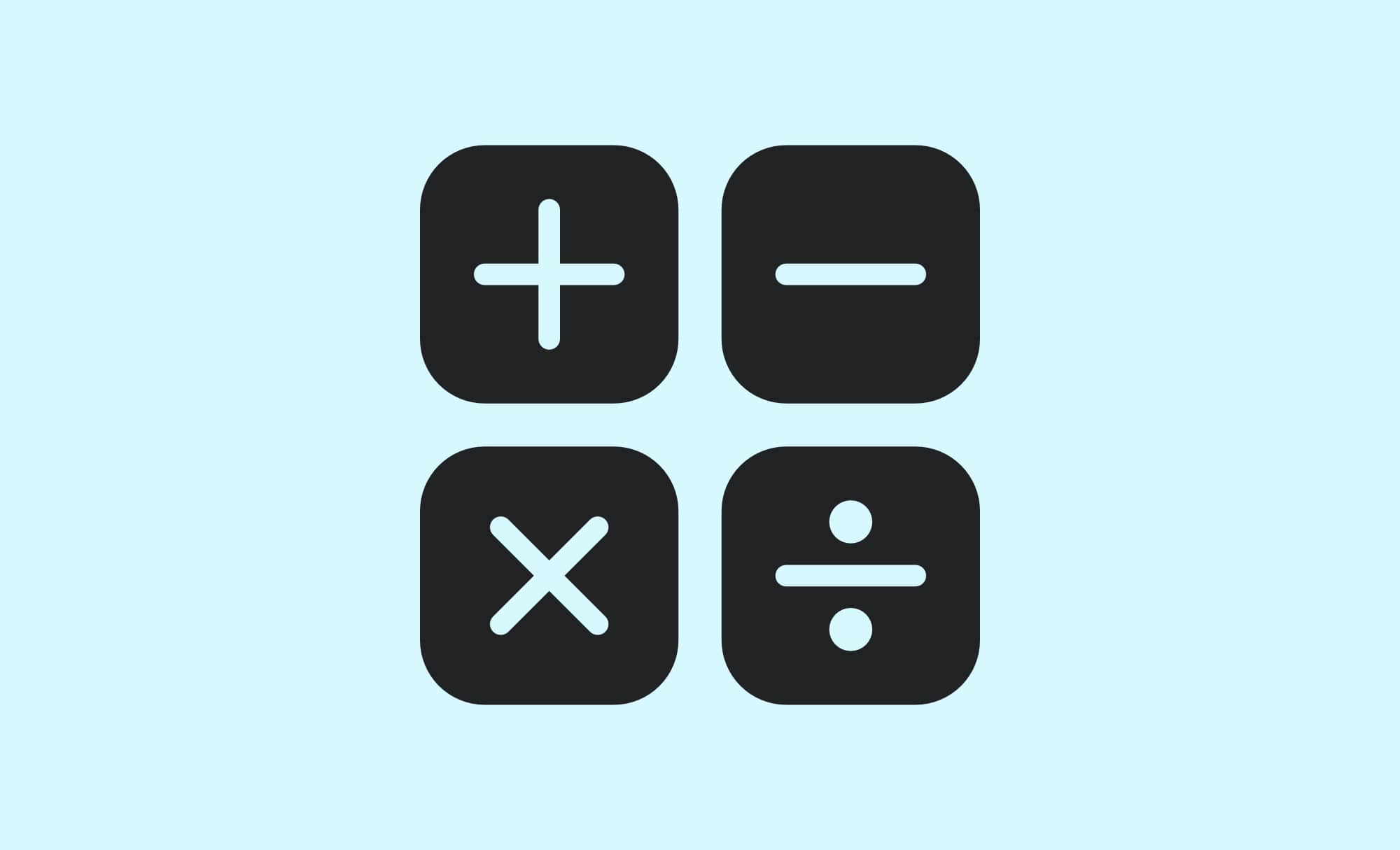# Maths74

GCSE Maths (Mathematics) learning topics and material for students in Year 7 to Year 11.

Check out the latest posts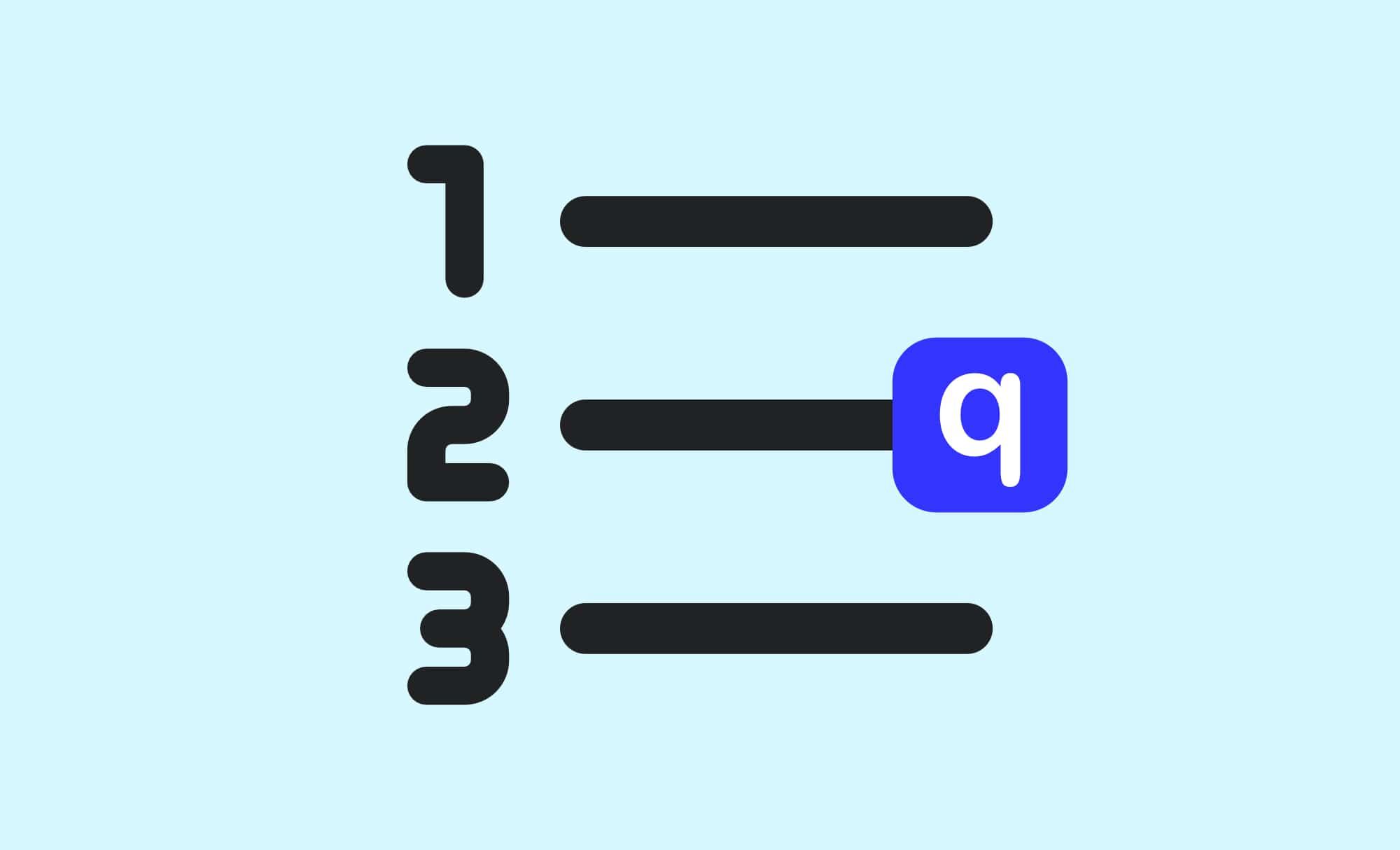## Types of Numbers Quiz

As various families have different members, so do differing numbers of the same family. Different types of numbers have been classified over the years. Use the quiz on this page to help you learn and remember the different types of numbers that exist in mathematics.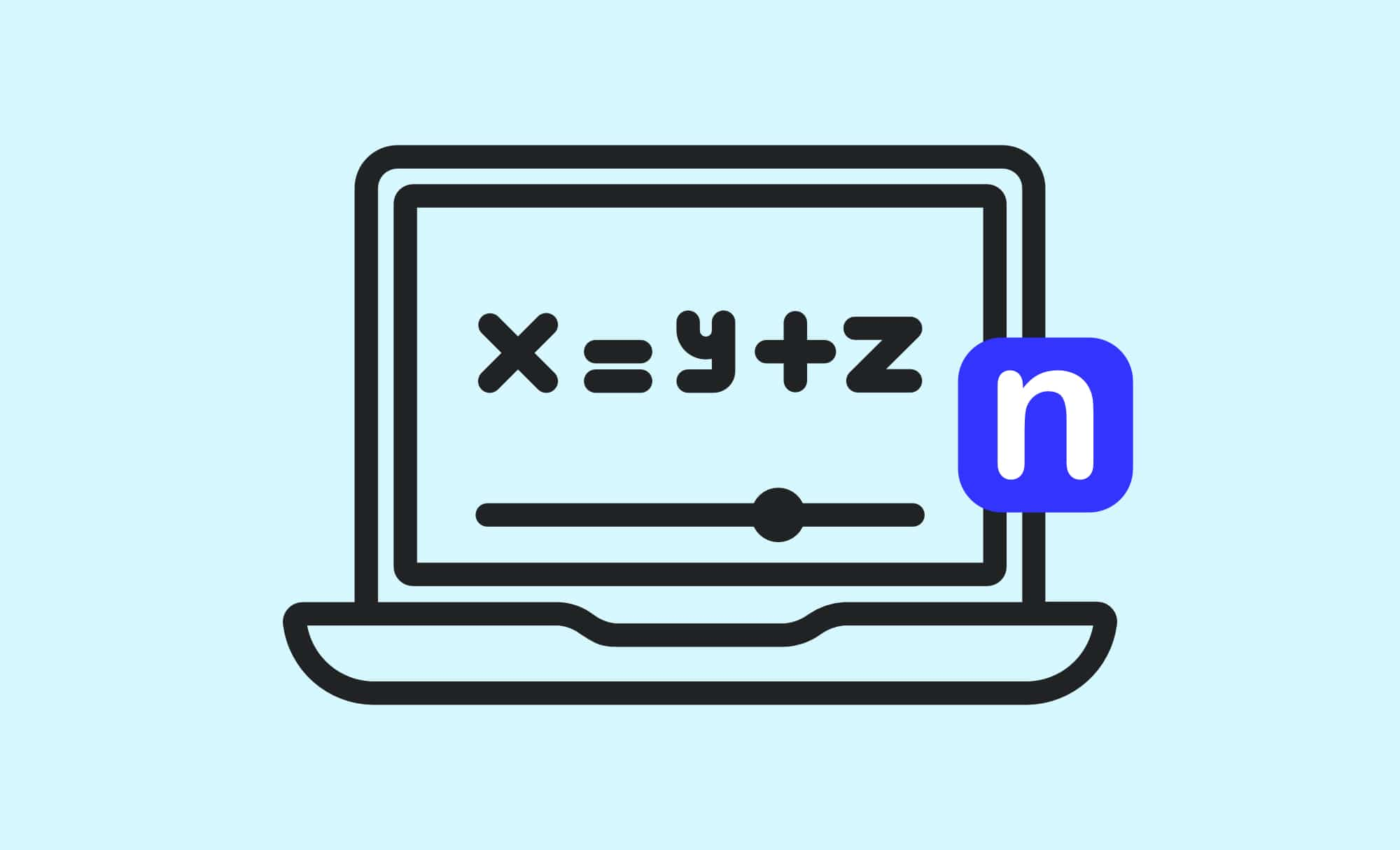## Simplifying Algebraic Equations

In order to understand anything in algebra, you have to have an understanding of what a term is. Terms are the building blocks of algebraic equations.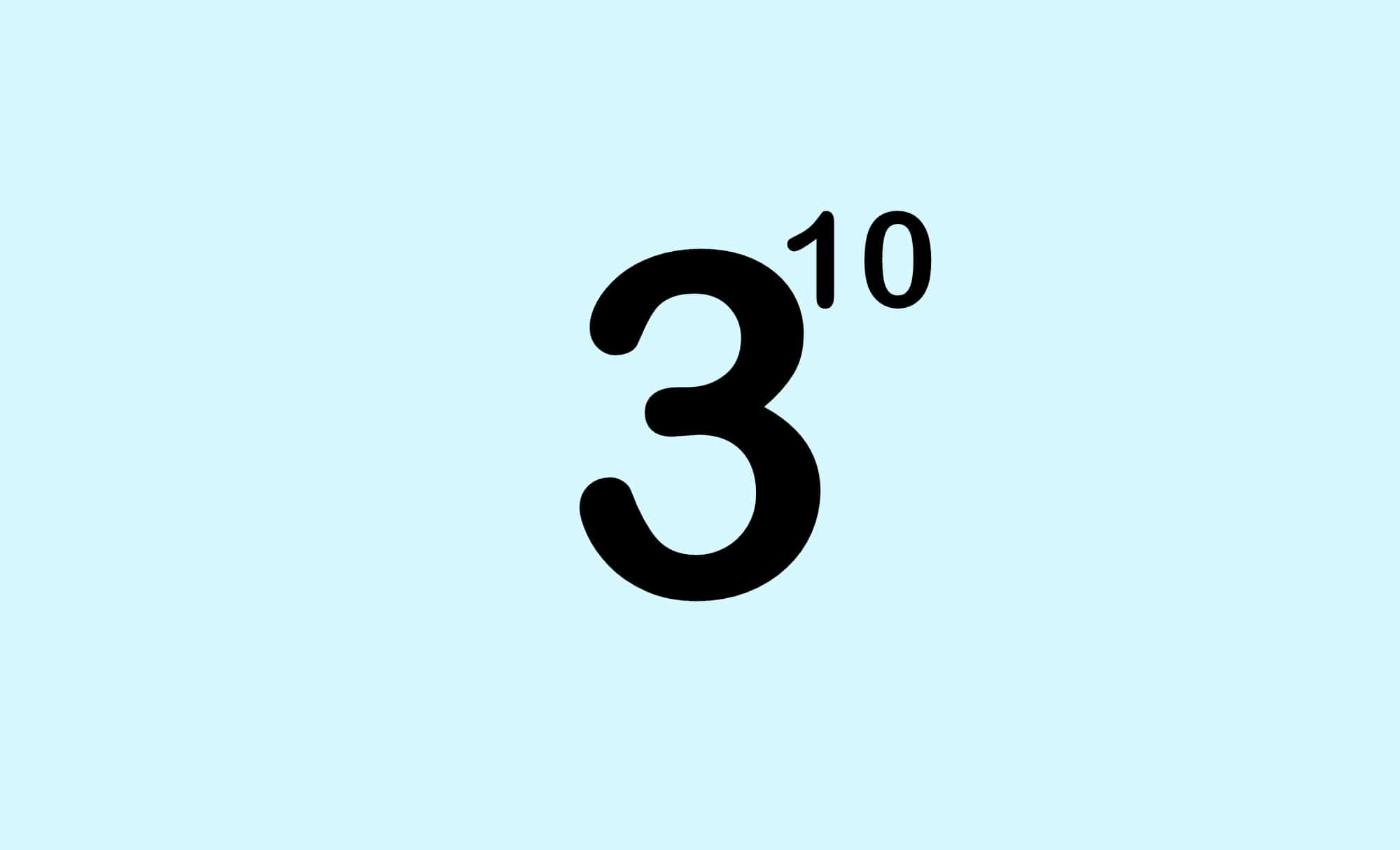## Writing Numbers including Standard Form P2

Calculations involving very large or very small numbers can be simplified by converting them into and out of standard form. A number can be converted into standard form by splitting it into two parts - a number between 1 and 10 multiplied by a power of 10.## Writing Numbers including Standard Form P1

Calculations involving very large or very small numbers can be simplified by converting them into and out of standard form. A number can be converted into standard form by splitting it into two parts - a number between 1 and 10 multiplied by a power of 10.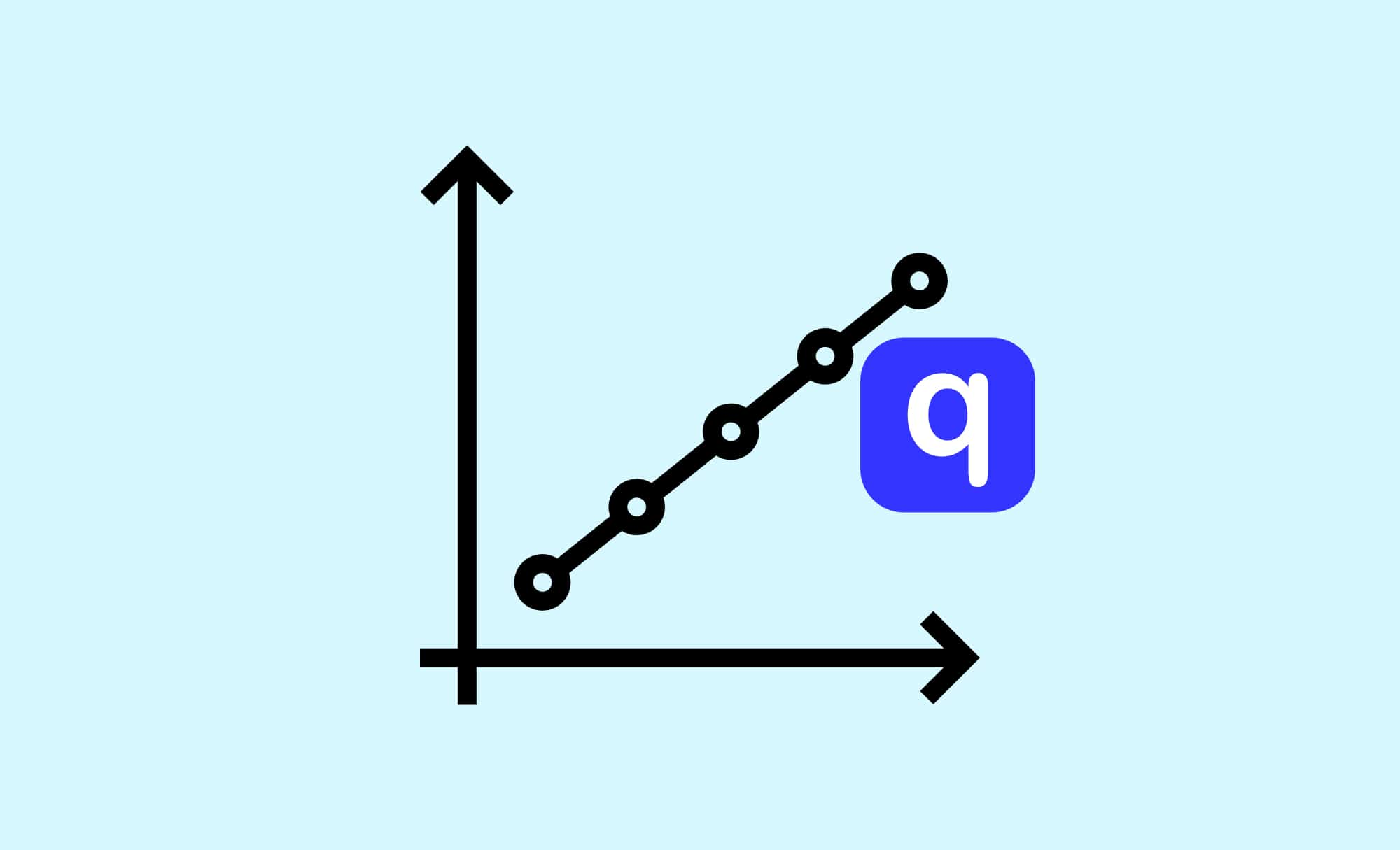## Direct & Inverse Proportion P2

The concept of proportion is used to show how quantities and amounts are related. Proportion rules govern how quantities change in relation to each other.## Direct & Inverse Proportion P1

The concept of proportion is used to show how quantities and amounts are related. Proportion rules govern how quantities change in relation to each other.

You’ve successfully subscribed to GCSE.CO.UK
Welcome back! You’ve successfully signed in.
Great! You’ve successfully signed up.